# GSEB Solutions Class 11 Chemistry Chapter 4 Chemical Bonding and Molecular Structure

Gujarat Board GSEB Textbook Solutions Class 11 Chemistry Chapter 4 Chemical Bonding and Molecular Structure Textbook Questions and Answers.

## Gujarat Board Textbook Solutions Class 11 Chemistry Chapter 4 Chemical Bonding and Molecular Structure

### GSEB Class 11 Chemistry Chemical Bonding and Molecular Structure Text Book Questions and Answers

Question 1.
Explain the formation of a chemical bond.
Atoms of the same or different elements combine resulting in the formation of a chemical bond between them. This formation of a chemical bond can be explained on the basis of various theories put forward from time to time.

The atoms of different elements combine with each other in order to complete their respective octets or duplet to attain stable inert gas configuration; This they do by either transference of electrons or by sharing of electrons. Another way to explain the formation of a chemical bond is it results in lowering of energy of the system and constituents (atoms) attain greater stability.Question 2.
Write Lewis dot symbols for atoms of the following elements: Mg, Na, B, O, N, Br.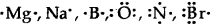Question 3.
Write Lewis symbols for the following atoms and ions: S and S2-, Al and Al3+; H and H.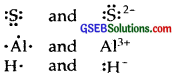Question 4.
Draw the Lewis structures for the following molecules and ions:
H2S, SiCl4, BeF2, CO2-3,HCOOH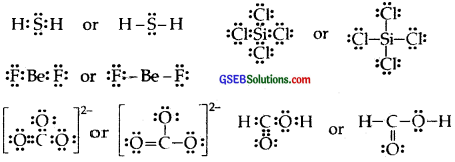Question 5.
Define the octet rule. Write its significance and limitations.
According to this rule: Atoms can combine either by transfer of valence electrons from one atom to another (gaining or losing) or by sharing valence electrons in order to have an octet (acquiring eight electrons) in their valence shells.

Significance: Acquiring 8 electrons (octet formation) in the valence shells of atoms leads to the stable electronic configuration because all noble gases (except He) have 8 electrons in their valence shells and they generally do not react chemically.
Neon = Ne = 10 = 2, 8
Argon = Ar = 18 = 2, 8, 8
Krypton = Kr = 36 = 2, 8; 18, 8
Xenon = Xe = 54 = 2, 8,18, 18, 8
Randon = Rn = 86 = 2, 8, 18, 32, 18, 8.
All other elements of the periodic table possess less than 8 electrons in their valence shells and hence are chemically reactive. Thus atoms of elements combine to acquire 8 electrons in their valence shells to acquire inertness or stability. the noble gas near to them.

Limitations : (1) Formations of compounds involving hydrogen. A hydrogen atom has only one electron in its valence shell, it needs one more electron to acquire the nearest noble gas configuration of Helium. Hence hydrogen needs to complete its duplet (acquiring 2 electrons in its valence shell) rather than an octet.

(2) Formation of compounds like BeCl2, BF3 AlCl3. In each of these examples central atom Be in BeCl2, B in BF3, and Al in AlCl3, has less than 8 electrons i.e., these compounds are electron-deficient compounds.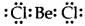[Be has only 4 electrons around it]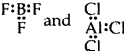[B and Al have only 6 electrons around it]
Thus octet rule is violated.

(3) Formation of compounds like PCls, SF6 IF7 In all these compounds, the central atom has more than 8 electrons.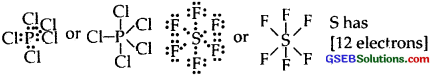[p has 10C]
I have 14 electrons around it in IF7

(4) Formation of compounds of noble gases. Noble gases on whom the octet rule is based have already 8 electrons in their valence shells and should not form compounds. Recently Xe has been found to form compounds like XeF2, XeF4, XeF6, etc.

(5) Odd electron bonds/Odd electron molecules. There are certain molecules and ions like NO, and $$\mathrm{O}_{2}^{-}$$ in which the bonded atoms contain an odd number of electrons between them:

• Nitric oxide [NO]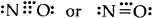• Superoxide ion ($$\mathrm{O}_{2}^{-}$$)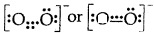(6) It does not explain the shape of the molecules and their relative stability.Question 6.
Write the favorable factors for the formation of an ionic bond.
The formation of an ionic bond would primarily depend upon.

1. The case of formation of the positive and negative ions depends upon the respective neutral atoms.
2. The arrangement of the positive and negative ions in the solid, i.e., of the lattice of the crystalline compound. These in turn depend upon the following.

(1) Low Ionization Enthalpy. The atom going to lose electrons to form a positive ion (cation) must lose them readily, i.e., the element must have low ionization enthalpy.
M(g) → M+(g) + e [Ionization enthalpy]
M must have low ionization enthalpy as mostly active metals like Na, K, Mg, Ca have.

(2) High negative electron gain enthalpy. The atom going to accept electrons must be able to retain it, i.e., it must have a high negative value of electron gain enthalpy,
X (g) + e → X (g) [Electron gain enthalpy]

(3) Enthalpy of lattice formation should be high. The energy released when The requisite number of positive and negative ions combine to form one mole of the ionic compound is called lattice energy or enthalpy.
The higher the value of lattice energy of the resulting ionic compound, the greater will be the stability of the compound, and hence the greater will be the case of formation.
M+ (g) + X (g) → MX (s) 1 Mole [Lattice enthalpy]

Question 7.
Discuss the shape of the following molecules using the VSEPR model :
BeCl2, BCl3, SiCl4, AsF5, H2S, PH3.
According to VSEPR theory: If the central atom is linked to different atoms or is surrounded by bond pairs as well as lone pairs of electrons, the repulsions between them are different. As a result, the molecule has an irregular or distorted geometry. The order of repulsions between electron pairs is as follows :
Lone pair-lone repulsion > lone pair-bond pair repulsions > bond pair-bond pair repulsions.
The exact shape of the molecule depends upon the total number of electron pairs present around the central atom.

BeCl2: Linear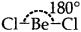[No lone pairs of electron on Be]

BCI3.: Trigonal planer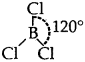[No lone pairs of electrons on B]

The central atom has Only 3 bond pairs of electrons.

SiCl4: Tetrahedral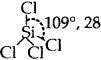[No lone pairs of electrons on Si]

Bond pairs of electrons with Si = 4.

AsF5: Trigonal bipyramid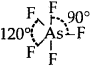[No lone pairs of electrons on As]

Bond pair = 5

The shape of H2S, The shape would have been tetrahedral if there were all bond pairs. But two lone pairs of electrons are present on sulfur and so the shape is distorted tetrahedral or angular.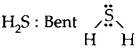Bond Paris = 2, lone pairs = 2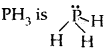distorted trigonal bipyramid/pyramidal
Had there been a bond pair of electrons in place of lone pair, the shape would have been tetrahedral but one lone pair causes repulsion and the angle between the bond pairs is reduced.Question 8.
Although geometries of NH3 and H2O molecules are distorted tetrahedral, the bond angle in water is less than that of NH3. Discuss.
Both NH3 and H2O involve sp3 hybridization and so their expected shape should be tetrahedral. But whereas in NH3, there is one lone pair of electrons on N, there are two lone pairs of electrons present on oxygen in H2O. According to VSEPR theory lone pair- lone pair repulsions, are more in H2O than lone pair-bond pair repulsions in NH3.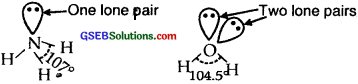The two lone pairs repel the bonded pairs of electrons more than one lone pair and therefore the bond angle between bond pair shrinks from 1070in the case of NH3 to 105.5° in H2O.

Question 9.
How do you express bond strength in terms of bond order?
Bond strength a Bond order.

Question 10.
Define the bond length.
The average distance between the centers of the nuclei of the two bonded atoms in a molecule is called it’s the bond length.

Question 11.
Explain the important aspects of resonance with reference to the CO2-3 ion.
The single Lewis structure based on the presence of two single bonds and one double bond between carbon and oxygen atoms is inadequate to represent the molecule accurately as it represents unequal bonds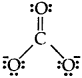According to the experimental findings, all carbon to oxygen bonds in CO2-3 are equivalent. Therefore the carbonate ion is best described as a resonance hybrid of the canonical forms I, II, and III shown below :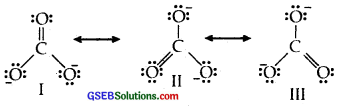Question 12.
H3PO3 can be represented by structures 1 and 2 shown below. Can these two structures be taken as the canonical forms of the resonance hybrid representing H3PO3? If not, give reasons for the same.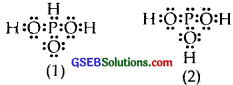No, these (1) and (2) structures cannot be taken as canonical forms of H3PO3 because the positions of atoms have been changed.Question 13.
Write the resonance structures for SO3 NO2 and NO3
Resonance structure of SO3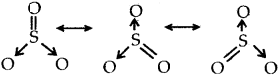Resonance structures of NO2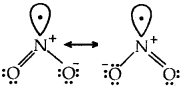Resonance structures of NO3 ion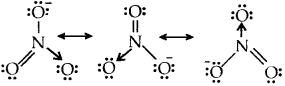Question 14.
Use Lewis symbols to show electron transfer between the following atoms to form cations and anions :

1. K and S
2. Ca and O
3. A1 and N.

(1) K and S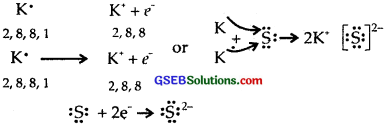(2) Ca and O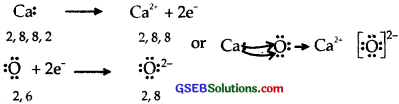(3) A1 and N.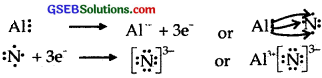Question 15.
Although both CO2 and H2O are triatomic molecules, the shape of the H2O molecule is bent while that of CO2 is linear. Explain this on the basis of dipole moment.
Both CO2 and H2O are triatomic molecules but the C02 molecule is linear whereas the H2O molecule is having a bent structure. H2O molecule has a dipole moment of 1.84 D. There are two O-H bonds in the H2O molecule and both the bonds are polar. Since the H2O molecule has a net dipole moment-, hence the two O-H dipoles are not in a straight line opposing each other (as they would cancel out in that case). This immediately rules out the linear structure (H-O-H) for water. The two O-H bonds cannot lie along the same line in the same direction also. Therefore, the two O-H bonds in the H2O molecule must be inclined to each other at a certain angle. Thus H2O molecule has an angular or bent structure.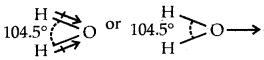CO2 has two C = O polar bonds. No doubt these polar bonds possess the same value of dipole moment, but the overall dipole moment of the molecule is found out to be zero. The explanation for this is individual dipole moments in this molecule are of equal magnitude but their directions are opposite to each other and hence cancel out. This shows that CO2 molecule is Linear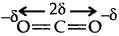Question 16.
Write the significance/applications of dipole moment.
Applications of Dipole moment:
1. In determining the polarity of bonds: As µ = e × d, obviously greater is the magnitude of dipole moment, higher will be the polarity of the bond. This is applicable to molecules containing only, one polar bond like HCl, HBr, etc. In non-polar molecules like H2, O2, N2, the dipole moment is zero. It is because there are no charge separations in these molecules [e = 0]. Thus dipole moment can also be used to distinguish between polar and non-polar molecules.

2. In the calculation of percentage ionic character.
Take the example of HCl. Its µ = 1.03 D
If HCl is 100% ionic, each end would carry charge = one unit i.e. 4.8 × 10-10 e.s.u.
d (bond length) in H – Cl = 1.275 Å
∴ for 100% ionic character, dipole moment will be
µionic = e × d = 4.8 × 10-10 e.s.u. × 1,275 × 10-8cm = 6.12 × 10-18 e.s.u cm = 6.12 D
∴ % ionic character = Img × 100 = $$\frac { 1.03 }{ 6.12 }$$ × 100 = 16.83.

3. In determining the symmetry (or shape) of the molecules. the Dipole moment is an important property in determining the shape ‘of molecules containing 3 or more atoms. For instant, if any molecule possesses two or more polar bonds, it will not be symmetric if it possesses some net molecular dipole moment as in the case of water (H2O) µ = 1.84 D and ammonia (µNH1 = 1.49 D). But if a molecule contains a number of similar atoms linked to a central atom, the overall dipole moment of the molecule are found out to be zero, this will imply that the molecule is symmetrical as is the case of CO2, BF3, CH4, CCl4, etc.

Question 17.
Define electronegativity. How does it differ from electron gain enthalpy?
Electronegativity of an element is the tendency of its atom to attract the shared pair of electrons towards itself in a covalent bond. It differs from electron gain enthalpy in the sense that whereas electronegativity is a property of an atom in a molecule, electron gain enthalpy is applicable to atoms in their isolated states.

Question 18.
Explain with the help of a suitable example polar covalent bond.
Polar covalent bond. Here the atoms in a covalently bonded molecule have unequal electronegativities. For example in HF, the electron pair is attracted more towards the F atom due to its higher electronegativity. HF may be written as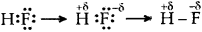where +δ refers to a small amount of positive charge and -δ refers to a small amount of negative charge.Question 19.
Arrange the bonds in order of increasing ionic character in the molecules: LiF, K2O, N2, SO2, and CIF3.
More the difference of electronegativity, more the ionic character of the molecules
N2 < SO2 < CIF3 < K2O < LiF

Question 20.
The skeletal structure of CH3COOH as shown below is correct but some of the bonds are shown incorrectly. Write the correct Lewis structure for acetic acid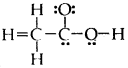The correct Lewis structure for acetic acid (CH3COOH) is :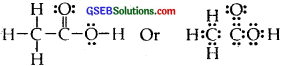Question 21.
Apart from tetrahedral. geometry, another possible geometry for CH4 is the square planer with the four H atoms at the corners of the square and the C atom at the center. Explain why CH4 is not square planar.
C = 6 = 1s2,2s2 2p1x 2p1y ground state
= 1s2, 2s1 2p1x2p|ly, 2p1z. excited-state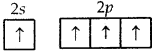C can undergo only sp3 hybridization which leads to tetrahedral geometry with 4 H atoms at the comers of tetrahedron and C in the center. Only these molecules can give rise to the square planar arrangement in which the central atom can undergo dsp2 hybridization which is not possible with C as it does not have a d orbital with it.Question 22.
Explain why the BeH2 molecule has a zero dipole moment although the Be-H bonds are polar.
Due to sp hybridization Shown by Be in BeH2, the molecule BeH2 is linear. Though the individual Be-H bonds are polar (due to the difference of electronegativity) yet BeH2 has a dipole moment of zero as dipole moment is a vector quantity (it has both magnitude . and direction). The individual polar character of the Be-H bond cancels out as the dipoles are equal and opposite as shown below :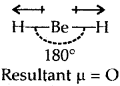Question 23.
Which out of NH3 and NF3 has a higher dipole moment and why?
The dipole moment of NH3 (1.46 D) is much higher than that of NF3 (0.24 D) Though the difference of electronegativity, between N and Fin NF3 [4.0 — 3.0 = 1.0] is nearly the same as. that between N and H in NH3 [N = 3.0 and H = 2.1; 3.0 – 2.1 = 0.9]
This i due to different directions of the bond moments of N-H and N-F bonds. In the first case, N is more electronegative but in the second case, F is more electronegative. Thus in NH3, the dipole moments of N-H bonds are in the same direction as that of .the lone pair (as shown in the diagram below).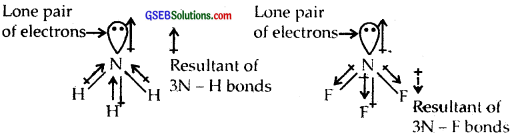Question 24.
What is meant by the hybridization of atomic orbitals? Describe the shapes of sp, sp2, sp3 hybrid orbitals.
Hybridization. It is defined as the mixing of the atomic orbitals belonging to the same atom but having slightly different energies so that redistribution of energy takes place between them resulting in the formation of new orbitals of equal energies and identical shapes. The new orbitals thus formed are known as Hybrid Orbitals.
sp Hybridisation. Here one and one p orbitals of the same atom mix up to give two sp hybrid orbitals with 1/2s and 1/2 p character and linear shape with a bond angle of 180° between them.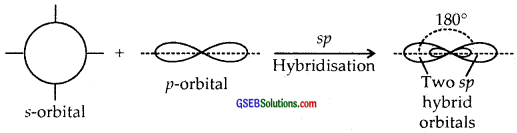sp2 Hybridisation. Here one and two p-orbitals of the same atom mix up to form three sp2 hybrid orbitals with 1/3 s and 2/3 p character.
They form Trigonal Planar shapes with an angle of 120° with themselves.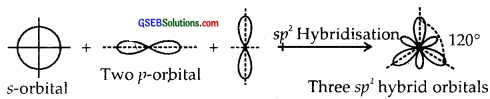sp3 Hybridisation. Here one and three p orbitals of the same atom mix up to give four sp3 hybrid orbitals with s-character and 3/4p character. They form tetrahedral shapes with 1/4 s-character and 3/4p character. They form tetrahedral shapes with angles of 109°, 28 with themselves.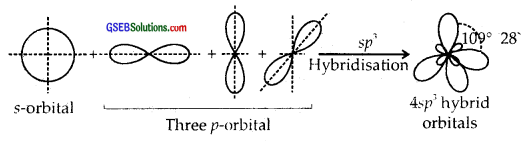Question 25.
Describe the change in hybridization (if any) of the Al atom in the following reaction.
AlCl2 + Cl → AlCl4
Electronic configuration of 13Al is 1s2 2s2 2p6, 3s23 p1x (ground state) and it is 1s2, 2s22p6, 3s1 p1x 3p1y (excited state). ∴ Al undergoes sp2 hybridization to form triangular planar geometry in AlCl3. To form A1C14, the empty 3pz is also involved so that hybridization is sp3 and the shape is tetrahedral.Question 26.
Is there any change in the hybridization of B and N atoms as a result of the following reaction?
BF3 + NH3 → F3B.NH3
In BF3, B is sp2 hybridized and in NH3, N is sp3 hybridized. After the reaction hybridization of B changes to sp3 but that of N remains unchanged.

Question 27.
Draw diagrams showing the formation of a double bond and a triple bond between carbon atoms in
(a) C2H2
(b) C2H4 molecules.
(a) Formation of a triple bond (lσ and two 2π bonds) in C2H2.
sp Hybridisation in C2H2: In the formation of ethyne – (C2H2) molecule, both the carbon atoms undergo sp-hybridization having two unhybridized orbital i.e., 2py and 2px.

On sp hybrid orbital of one carbon atom overlaps axially with sp hybrid orbital of the other carbon atom to form C-C sigma bond, while the other hybridized orbital of each carbon atom overlaps axially with the half-filled s orbital of hydrogen atoms forming two C-H σ bonds. Each of the two unhybridized p orbitals of both the carbon atoms overlaps sidewise to form two π bonds between the carbon atoms. So the triple bond between the two carbon atoms is made up of one sigma and two pi bonds as shown in Fig. below
(a) Formation of a bonds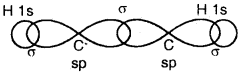(b) Formation of 2π bonds in C atoms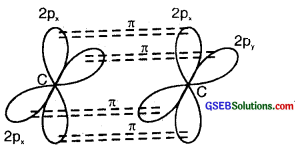(c) Formation of a triple bond carbon atoms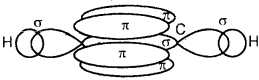(b) Formation of a double bond (lσ and 1π) in C2H4)
sp2 Flybridisation in C2H4: In the formation of an ethane (C2H4)molecule, one of the sp2 hybridized orbital of another carbon atom to form a C-C sigma bond. While the other two sp2 hybrid orbitals of each carbon atom are used for making sp2 – s sigma bonds with two hydrogen atoms.

The unhybridized orbital (2p) of one another atom overlaps sidewise with the similar orbital of the other carbon atom to form a weak π bond, which consists of two equal electron clouds distributed above and below the plane of carbon and hydrogen atoms.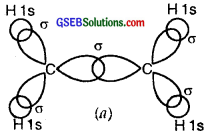Thus, in the ethene (C2H4) molecule, the carbon-carbon bond consists of one sp2-sp2 sigma bond and one pi (π) bond between p orbitals which are not used in the hybridization and are perpendicular to the plane of the molecule; the bond length 134 pm. The C-H bond is sp2-s sigma with a bond length 108 pm. The H-C-H bond angle is 117.6° while the H-C-C angle is 121°. The formation of sigma and pi bonds in ethane is shown in Fig.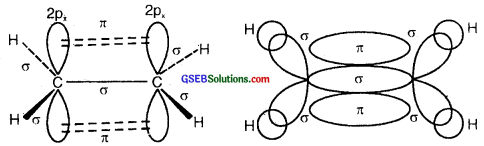(b) and (c) Formation of one n bond between two C atoms.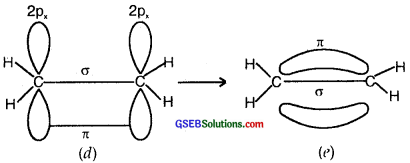(d) and (e) Formation of a double bond (one σ; one π) between two C atoms.

Question 28.
What is the total number of sigma and pi bonds in the following molecules?

1. C2H2
2. C2H4

1. There are a total of three sigma bonds (one C-C sigma and two C-H sigma) and Two pi bonds (between the two C atoms) in C2H2.
2. There are a total of five sigma bonds (one C-C sigma and four C- H sigma) and only one pi bond (between the two C atoms) in C2H2.Question 29.
Considering the x-axis as the internuclear axis which out of the following will not form a sigma bond and why?

1. 1s and 1s
2. 1s and 2px
3. 2py and 2py
4. 1s and 2s.

3. Since A-axis is being considered as the internuclear axis and the y-axis is perpendicular to A-axis, therefore 2py and 2py atomic orbitals can overlap only side-wise (lying on the y-axis). Since overlapping of y-orbitals side-wise results in the formation of pi (π) bond, therefore 2py and 2py will not form a sigma bond.

Question 30.
Which hybrid orbitals are used by carbon atoms in the following molecules?

1. CH3 – CH3;
2. CH3 – CH=CH2;
3. CH3 – CH2 – OH;
4. CH3 – CHO
5. CH3COOH

1. In CH3 – CH3 carbon uses sp3 hybrid orbitals.
2. In CH3 – CH = CH2; carbon used sp2 and sp3 hybrid orbitals.
3. In CH3 – CH2 OH; carbon uses sp3 hybrid orbitals.
4. In CH3 – CHO carbon uses sp2 and sp3 hybrid orbitals.
5. In CH3COOH carbon uses sp2 and sp3 hybrid orbitals.

Question 31.
What do you understand by bond pairs and lone pairs of electrons? Illustrate by giving one example of each type.
The electrons which are present between the two atoms and shared by them are called bonded pairs of electrons. For example, an H2 molecule has only a bond pair of electrons. Whereas NH3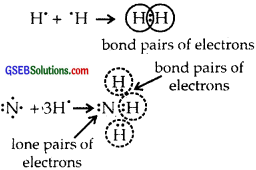the molecule has both lone pairs (as shown above) and three bond pairs of electrons. H2O has two lone pairs of electrons. Lone pair of electrons are those which are not shared by another atom.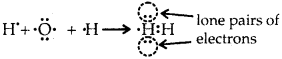Question 32.
Distinguish between sigma and a pi bond.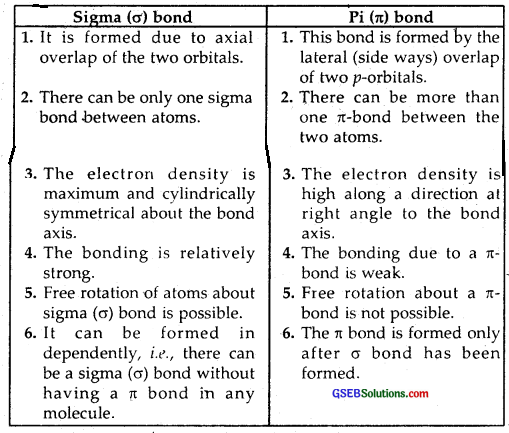Question 33.
Explain the formation of H2 molecule on the basis of valence bond theory.
In H2 molecule 1s atomic orbitals of both the hydrogen atoms overlap end on (s-s overlap) resulting in the formation of a. sigma bond, between them.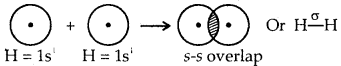Question 34.
Write the important conditions required for the linear combination of atomic orbitals to form molecular orbitals.
The linear combination of atomic orbitals to form molecular orbitals takes place only if the following conditions are satisfied.

1. The combining atomic orbitals must have the same or nearly the same energy:
This means that 1s orbital because the energy of 2s orbital is applicably higher than that of 1s orbital. This is not true if the atoms are very different.

2. The combining atomic orbitals must have the same symmetry about the molecular axis. By convention, the z-axis is taken as the molecular axis. It is important to note that atomic orbitals having the same or nearly the same energy will not combine if they do not have the same symmetry. For example, the 2pz orbital of one atom can combine with the 2pz orbital of the other atom but not with 2px or 2py orbitals because of their different symmetries.

3. The combining atomic orbitals must overlap to the maximum extent. The greater the extent of overlap, the greater will be the electron- density between the nuclei of a molecular orbital.Question 35.
Use molecular orbital theory to explain why the Be2 molecule does not exist.
The electronic configuration of Be = 4 = 1s2, 2s2.
The molecular orbital (hypothetical) is KK[σ2s]2[σ*2s]2
Bond order = $$\frac { 4 – 4 }{ 2 }$$ = 0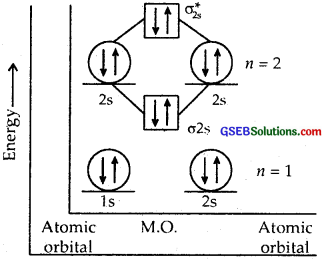Question 36.
Compare the relative stability of the following species and indicate their magnetic properties
O2, $$\mathrm{O}_{2}^{+}$$, $$\mathrm{O}_{2}^{-}$$, (sujjeroxide) $$\mathrm{O}_{2}^{2-}$$ (peroxide)?
Molecular orbitals of O2, $$\mathrm{O}_{2}^{+}$$, $$\mathrm{O}_{2}^{-}$$, and $$\mathrm{O}_{2}^{2-}$$ are as follows:
O2 : (σ1s)2 (σ*1s)2 (σ2s)2 (σ*2s)2 (σ2pz)22py)2 (π*2px)1 = (π*2py)1
Bond order = $$\frac { 1 }{ 2 }$$ (10 – 6) = $$\frac { 4 }{ 2 }$$ = 2; It has two unpaired electrons one in each of π*2px and π*2py molecular orbitals. Therefore, it is paramagnetic

$$\mathrm{O}_{2}^{+}$$ : (σ1s)2 (σ*1s)22s)2
(σ*2s)22pz)2 = (π2py)2 (π*2px)1
Bond order = $$\frac { 1 }{ 2 }$$ (10 – 7) = $$\frac { 3 }{ 2 }$$ = 1$$\frac { 1 }{ 2 }$$

There is one unpaired electron present in π*2px MO.
∴ $$\mathrm{O}_{2}^{-}$$ is also paramagnetic like $$\mathrm{O}_{2}^{+}$$, but less paramagnetic than $$\mathrm{O}_{2}^{-}$$
$$\mathrm{O}_{2}^{2-}$$ : (σ1s)2 (σ*1s)22s)2 (σ*2s)22pz)22pz)22px)2 = (π2py)2 (π*2px)2 = (π*2py)2
Bond order = $$\frac { 1 }{ 2 }$$ (10 – 8) = $$\frac { 2 }{ 2 }$$ = 1
There is no unpaired electron present in $$\mathrm{O}_{2}^{2-}$$
∴ $$\mathrm{O}_{2}^{2-}$$ is diamagnetic.
Thus O2 is more paramagnetic than $$\mathrm{O}_{2}^{+}$$ or $$\mathrm{O}_{2}^{-}$$ whereas $$\mathrm{O}_{2}^{2-}$$ is diamagnetic.
The stability of these species varies directly as bond order
∴ Stability increases from $$\mathrm{O}_{2}^{2-}$$ to $$\mathrm{O}_{2}^{+}$$ follows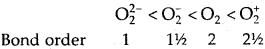Question 37.
Write the significance of plus and a minus sign shown in representing the orbitals.
Crests of the electron wave are usually given as ‘+’ sign and the trough a ‘-‘ sign. Thus bonding MO is formed by the combination of ‘+’ with ‘+’ and ‘-‘ with ‘-‘ part of the electron wave whereas antibonding MOs are formed by the overlap of ‘+’ with ‘-‘ part. These ‘+’ or ‘-‘ signs have nothing to do with the electrical charges on the orbitals.

Question 38
Describe the hybridization in the case of PCl5. Why are the axial bonds longer as compared to equatorial bonds?
Z of P in PCl5 = 15 = 1s2, 2s2 2p6, 3s2 3p3 ground state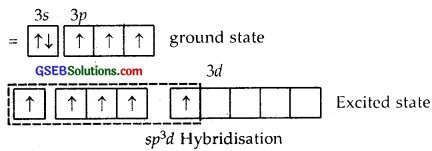Here one 3s, three 3p and one 3d [3d2z] atomic orbitals undergo hybridization giving to sp3d hybridization.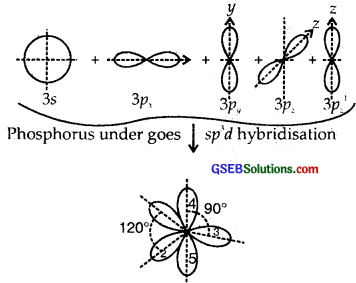1, 2, 3 are inclined at angles of 120°, 4, 5 are the inclined ⊥ plane of the paper.
The shape of PCl5 is Trigonal Bipyramid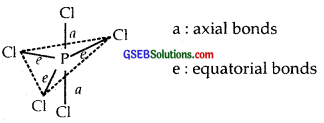The P-Cl equatorial bond length is 2.04 Å whereas the P-Cl axial bond length is 2.10 Å. The reason for the longer axial bond length is that the three P-Cl equatorial bonds are repelled only by two electron pairs. Thus the repulsion exerted on the axial bond pairs is more and hence bond length is longer.

Question 39.
Define hydrogen bond. Is it weaker or stronger than the van der Waals forces?
When a molecule containing an H atom is linked to a highly electronegative atom (like N, O, F), this atom attracts the shared pair of electrons more and so this end of the molecule becomes a slightly negative pole of the other molecule and so on and as a result, a weaker bond is formed between them. This bond is called a hydrogen bond. It is represented by dotted lines as shown below :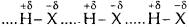As a result of Hydrogen bonding, an H-atom links the two electronegative atoms simultaneously, one by a covalent bond and the other by a hydrogen bond Hence it is said to form a Hydrogen bridge. A hydrogen bond is a weak bond, but it is stronger than van der Waals forces.Question 40.
What is meant by the term bond order ? Calculate the bond order of : N2, O2, $$\mathrm{O}_{2}^{+}$$ and $$\mathrm{O}_{2}^{-}$$.
Bond order = $$\frac { 1 }{ 2 }$$ (Nb – Na), where Nb = number of electrons
Bond orders of N2, O2, $$\mathrm{O}_{2}^{+}$$ and $$\mathrm{O}_{2}^{-}$$.
Bond order of O2 = $$\frac{\mathrm{N}_{b}-\mathrm{N}_{a}}{2}$$ = $$\frac{10 – 6}{2}$$ = $$\frac { 4 }{ 2 }$$ = 2
Bond order of $$\mathrm{O}_{2}^{+}$$ = $$\frac{\mathrm{N}_{b}-\mathrm{N}_{a}}{2}$$ = $$\frac{10 – 5}{2}$$
= 2$$\frac { 1 }{ 2 }$$
M.O. of $$\mathrm{O}_{2}^{+}$$ : KK (σ2s)2 (σ*2s)22pz)22px)2 = (π2py)2 (π*2px)1
M.O. of $$\mathrm{O}_{2}^{-}$$ : (σ2s)2 (σ*2s)22pz)22px)2 = (π2py)2 (π*2px)2 = (π*2py)1
Bond order of $$\mathrm{O}_{2}^{-}$$ = $$\frac{\mathrm{N}_{b}-\mathrm{N}_{a}}{2}$$ = $$\frac{10 – 7}{2}$$ = $$\frac { 3 }{ 2 }$$
Thus Bond order of N2 = 3; O2 = 2, $$\mathrm{O}_{2}^{+}$$ 2$$\frac { 1 }{ 2 }$$; $$\mathrm{O}_{2}^{-}$$ = 1$$\frac { 1 }{ 2 }$$.